# The correct way to tune parameters ? Part 1 — Optimizer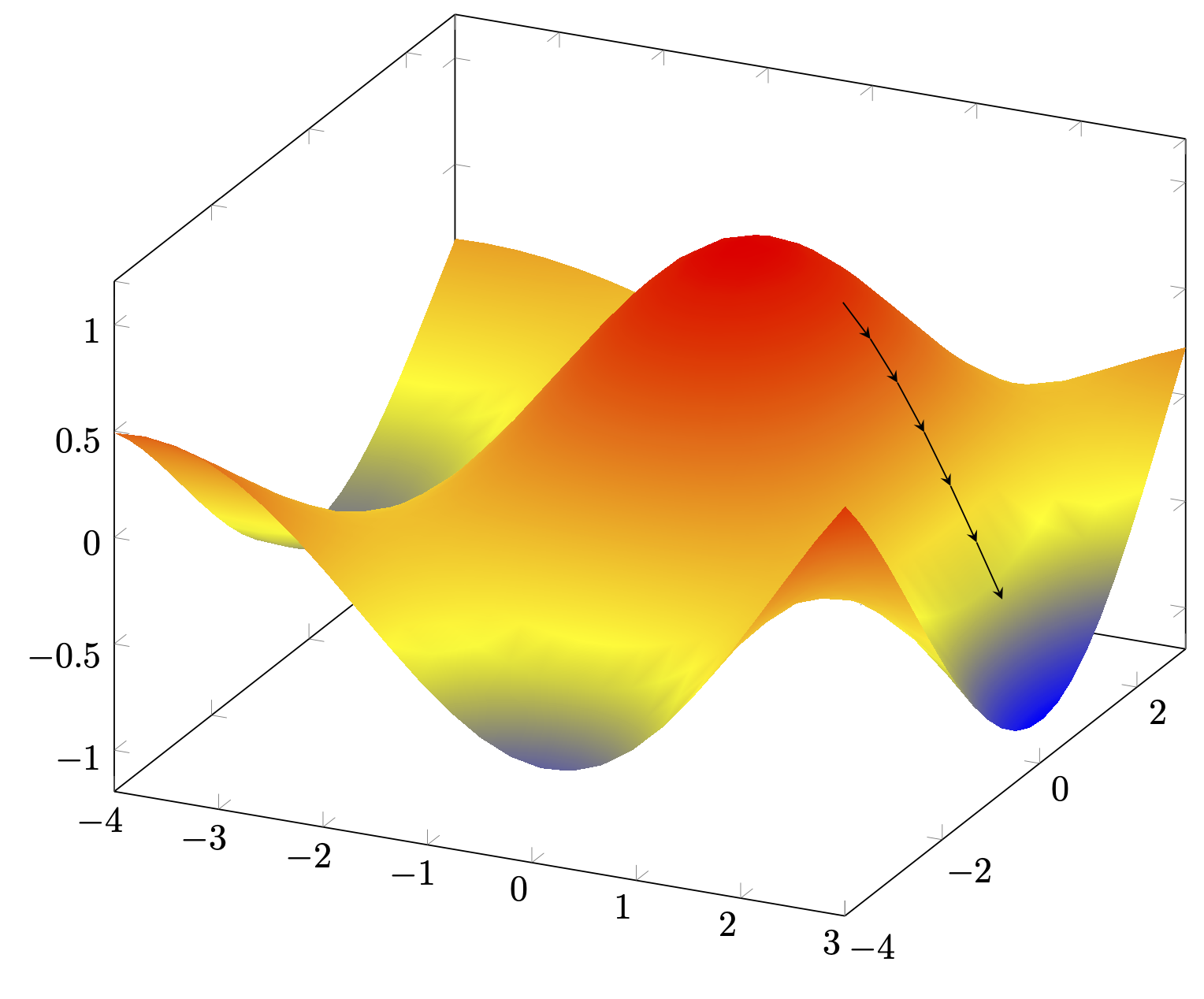This is the first article on my series about Hyper-parameter Tuning for object detection. It may seems like a basic topic to some of you but sometimes it’s good to cover the basics again !

I will release one article a week with (approximately) this schedule :

1. Part 1 : Optimizer
2. Part 2 : Batch size
3. Part 3 : Learning rate
4. Part 4 : Input shape
5. Part 5 : Data Augmentation

When you want to train your own Object Detection model, you will find hundreds of different libraries, frameworks, or Github repos that would do the job.

• Tensorflow Object Detection API
• YOLO v1/2/3/4/5/5000/hakimbo…
• Pytorch
Problem is, you can’t just test them all, it takes a lot of time and computing power to train that much models.

Problem is, you can’t just test them all, it takes a lot of time and computing power to train that much models.

A config file (usually named pipeline.config) is the place where all the hyper-parameters needed for training and evaluation are. There you will be able to define everything, from the path of the base checkpoints to the number of epochs you want to train.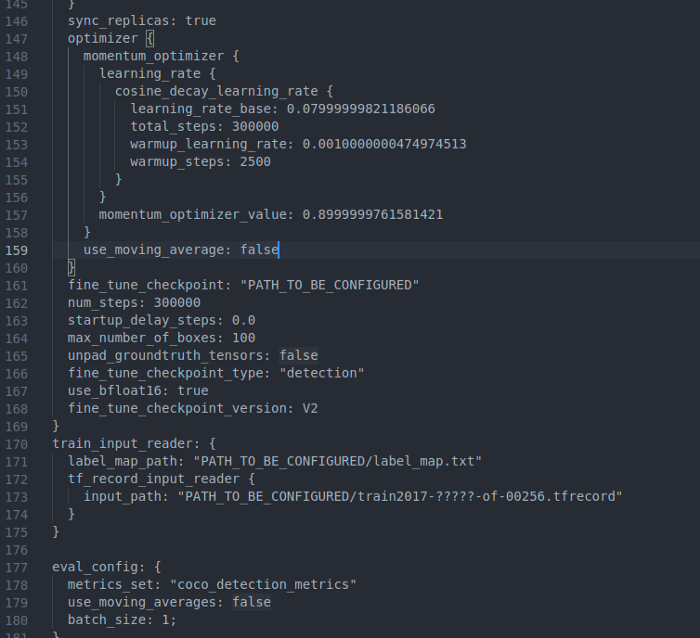As you can see there is a lot of parameters that you can edit, some of them you may have heard about like ‘learning_rate’ or ‘num_steps’ but also other where you will not know what to write for.

But don’t worry, there is only a few parameters that we actually do need to update. Indeed, most of the parameters such as the architecture definition has already been optimized by the Tensorflow teams.

If you start training without tuning much parameters, you will definitely have some results, but at some point, you’ll have a hard time increasing the performance of your model.

What you need to do is called Hyper-parameter Optimization.

You may have had the feeling that you never know for sure what parameter has the most influence on the training and evaluation metrics, because you don’t understand them deeply and also because you can’t really test every parameter combination (grid-search) because it’s too computationally expensive.

In this article series, we will study the most important parameters and their influence on training.

## This article will be dedicated to the choice of the optimizer !

Here is a little summary of what you can expect in this article :

1. A reminder on Optimization Problems
2. Theoretical study of each Optimizer
3. Practical differences on a real Computer Vision use-case
4. Conclusion

In the Tensorflow Object Detection API, we have to choose our optimizer within the following :

• Momentum Optimizer
• RMS_Prop Optimizer

We will try training the same model with the same parameters except the optimizer so we can objectively compare its influence on the training.

For our test, we will train an EfficientDet-d0.
We will use Picsellia's Platform for the setup and training so we can focus on our study.

But first, a bit of theory to truly understand what is behind each optimizer.

## An optimization problem

Optimizer are called optimizer because they try to solve an optimization problem. You might (I hope so) have already seen some curves like this one :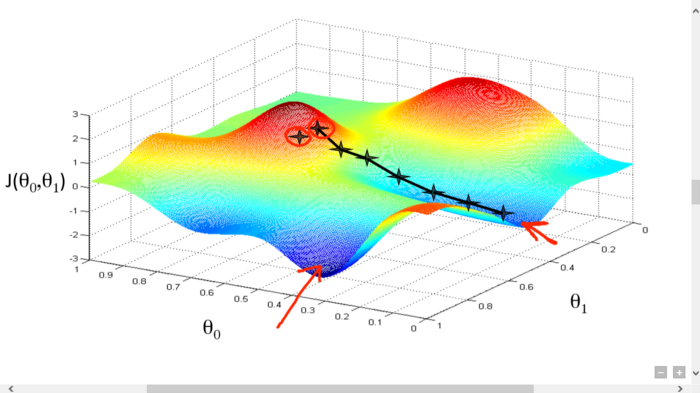This is the a 2D projection of our loss function for every values of two parameters.

Our goal, when we train a neural network, is to find the optimal values of each and every parameter so we find a minima in our loss function. But we can’t just try every possible combination of parameters, it is way too greedy and inefficient.

That’s why advanced optimization techniques such as gradient descent are used in neural networks.

As an ML practitioner, you must know that deep learning relies heavily on gradient descent to optimize the weights and biases of our neural networks.

In its original form, the equation of update during back-propagation looks like this :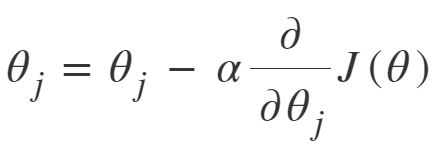Where θj is the weight value for iteration j, α is the learning rate and J(θ) is the value of the cost function (in our case the loss function).

This is just one GD iteration, now we have to repeat this operation until our loss function reach (hopefully) a global minima.

But this simple gradient descent only takes into account the derivative of the loss function at the last iteration and in cases like below, this can lead to some problems.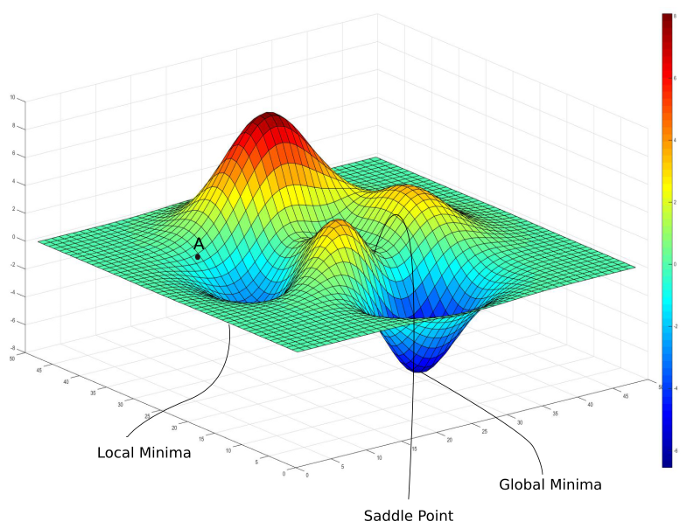To fight against local minima and saddle points, some randomness has been added to the equation in the name of SGD (Stochastic Gradient Descent). This means that instead of computing the gradients at each iterations for all training samples, we will choose samples randomly at each step so we can escape those uncomfortable situations.

But the problem that remains unsolved is the one of pathological curve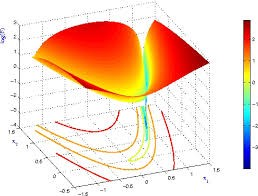To go to the minima we are looking for, we have to go through a ‘ravine’ (green part) which is what we call a Pathological Curve. I’ll will not dive deep into this concept in this article but let just say that it’s this particular problem that led to a need in different optimizer than standard gradient descent which we are going to study in this article.

To know more about those optimization techniques, with more drawing, I suggest you read this really complete article from Paperspace here.

## Momentum Optimizer

The idea behind Momentum is that the information given by the gradient for a single step is not enough, and that some second order approach (like Newton’s Method) could help us get out of convergence traps.

What it actually does is that it accumulates the gradient of the previous steps to determine which direction to search for our minima. Here are the new equations :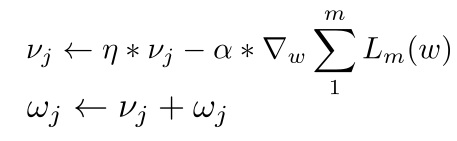The first equations where the gradient calculation takes place is composed of two parts, the first one is the accumulation of the gradient over steps, with η the value of the momentum, and the second one is our main gradient term.

This means that over time, we are taking an exponential average of the gradient steps, the most recent term is weighted with η, the second with η squared, the third with η cubed etc… (as shown in the following equations with a momentum value of 0.9)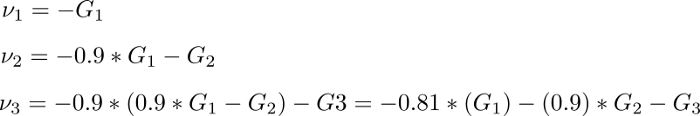As we can see, the importance of each gradient step in the computation of the actual step is decreasing over time.

But how does this helps us get out of the mighty pathological curve ?

Well if we project ourselves in a simpler case where each gradient update is resolved into components in only two axes w1 and w2 like below. As the normal path is kind of a zig-zag between the edges of our ‘ravine’ (the sign of the gradient along w1 is changing at each step), we can intuit that, by taking into account the previous gradient steps, we will kind of cancel the gradient in the w1 direction which will lead to faster convergence in the direction of our minima, w2.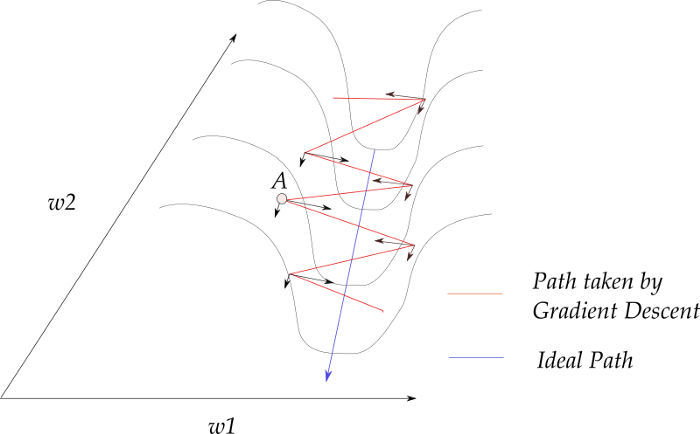## RMSProp Optimizer

Root Mean Square Propagation (RMSProp) is an optimizer that leverages the momentum concepts that we saw earlier while adding some adjustments.

The main difference with the Momentum Optimizer is that RMSProp chooses a different learning rate for each parameter and the update is consequently done for each parameter separately according to the following equations.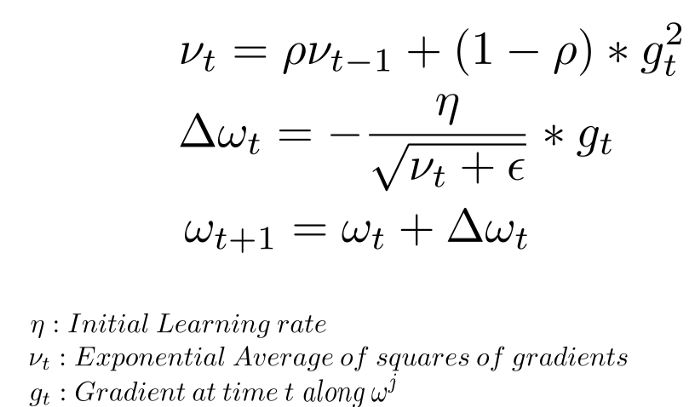Because of that, the gradient gt here corresponds to the component of the gradient along the direction represented by the parameter we are actually updating.

The first equation looks a lot like momentum, indeed we are still computing an exponential average of our gradient, except that this time, the gradient of the current step is squared.

If we look back to our pathological curve diagram, as the gradients along the w1 component are much larger than the ones along w2, this time they will not cancel out as we are squaring them and adding them.

The parameter ρ is usually set to be 0.9 but can be tuned.

The second equation is the computation of the step size, η is our initial learning rate (chosen by us) and is divided by our the exponential average we just calculated. Following our example, since the average along w1 is way more large than it is for w2, the step size along w1 will then be way smaller than the one along w2. Hence helping us avoid bouncing between the ridges and moving towards the minima.

The parameter ε is just here to ensure that we will never divide by 0 in the second equation, that’s why it’s really small (~1e-10).

The third equation is the classical update step.

Now that we have learned Momentum and RMSProp, we can finally understand what is really behind Adaptative Moment Optimization (Adam).

What Adam does is actually combining the heuristics of both methods, this gives us the following equations.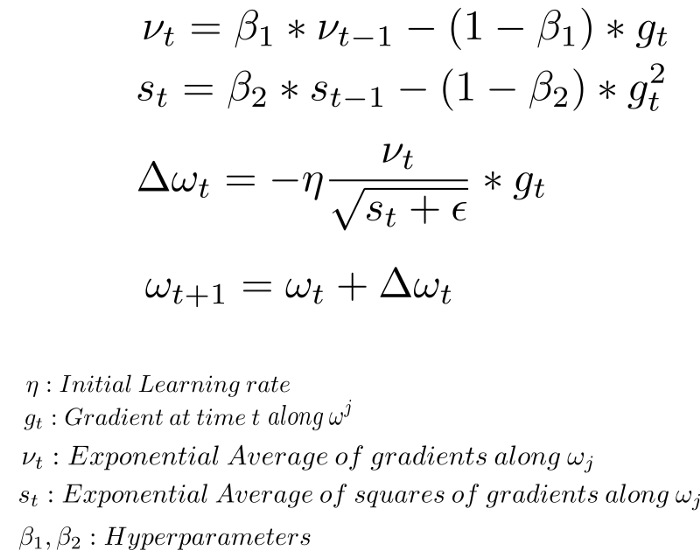Let’s stick one last time to our pathological curve diagram.

Here we can clearly see that νt is given by the momentum formula (gives a tendency to zero the gradient of the w1 component) and st is given by RMSProp (decrease the learning rate for huge gradients along the w1 component).

This gives us a combined step size in the second equation and the third equation is as always the update step.

The hyperparameter β1 is generally kept around 0.9 while β2 is kept at 0.99. Epsilon is chosen to be 1e-10 as for RMSProp.

While we might think now that Adam should be the best performing because it’s the combination of the best of both Momentum and RMSProp, we will see that there is a different kind of truth in practice.

## Testing all the optimizer

For the sake of test, we used a dataset ready for object detection containing two classes, car and pedestrian, with respectively 145k and 106k objects.

The training images looks like this.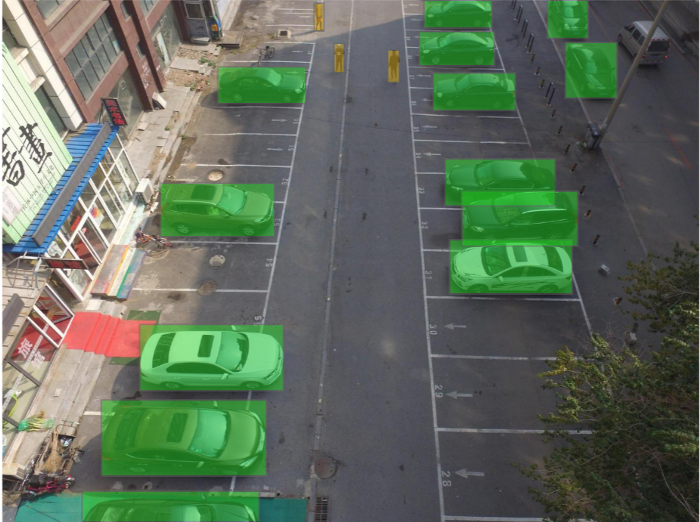You can find this dataset and many others ready for training on Picsellia.

Now I will set up some experiments using a pre-trained EfficientDet-d0 architecture. I’ll also define the same parameters for every experiment except the optimizer choice that will be different so we can objectively compare its influence on training.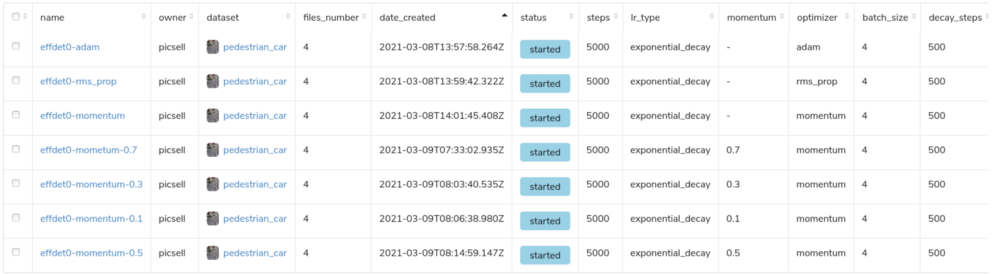Thanks to Picsellia, now I just have to launch these experiments with the click of a button and wait for them to finish.

As the training will take place on NVIDIA V100S, and that we set our number of steps to 5000, it should not take long.

Let’s compare our first three experiments, we will talk about the other later.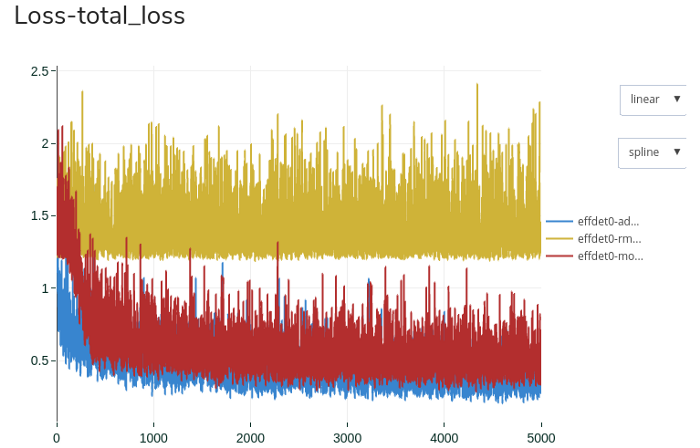If we look at the loss (remember that it’s the function for which we are looking for the minima), we can clearly see 3 different behaviors.

Please don’t mind the noise, this has to do with the dataset we are using, I’ll try to use another one (simpler) next time.

What does this plot tells us about our optimizer ?

• RMSProp seems to not converging at all
• Adam converge faster and to an average lower value
• Momentum does converge but slower than Adam
It seems that, as we could have predict from the theory explained earlier, that the Adam Optimizer is the best performing one.

For the rest of our study we will not keep RMSProp as it doesn’t perform well and it might be because of other parameters.

Now we will check the influence of the momentum value on the training for the Momentum Optimizer and then compare all of this with Adam. The original training with the Momentum Optimizer has a default momentum value of 0.9.

The optimum value will vary within the 0.1–0.9 range with a 0.2 step.

You might ask why we don’t change the momentum value for Adam as it is derived from the Optimum optimizer ?

The answer is simple : You can’t do it with the Tensorflow Object Detection API. I suppose that this is because it is automatically tuned and that changing it would cause more harm that good but I don’t really have the answer by now…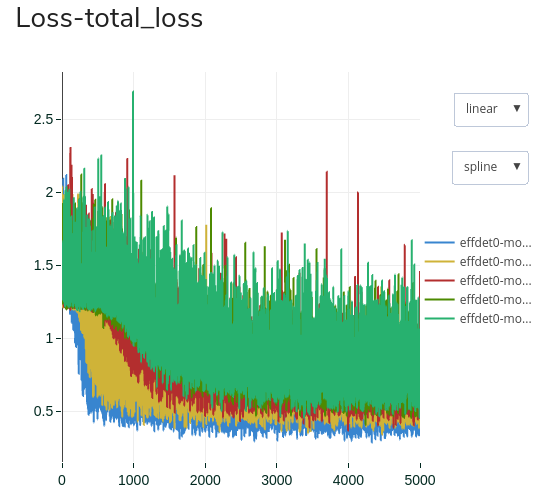Here is the superposition of our loss curves using only the Momentum Optimizer with a varying momentum.

Here are the legends :

• Pure Blue — momentum = 0.9
• Yellow — momentum = 0.7
• Red — momentum = 0.5
• Olive Green — momentum = 0.3
• Turquoise — momentum = 0.1

What we can conclude is that, in our case, the bigger the momentum, the faster the convergence and the lower the minima. It’s almost like the default values we spoke about earlier has been wisely chosen 😉

## Conclusion

• Explaining what hyper-parameters are and how they should influence training ;
• Showing you how to tune them wisely and see how they actually influence training ;
• Conclude on if and how some parameters needs to be tuned or not.

Today we made an in-depth study on deep-learning optimizers, it’s always good to remember what the most basics concept are and how they led to the architecture and techniques we see today.

What we can say about our study is that :

• You shouldn’t train a model with an RMSProp optimizer unless you are absolutely sure that your other hyperparams are perfectly tunes (and you’re mostly not).
• If you train with an Optimum optimizer, don’t bother training with momentum values below 0.7 (even 0.8), and always start from the highest value and decrease if you think you must.
• But always try to at least train one time with Adam optimizer and compare it with your best training with the Optimum optimizer, it will be the best performer in many cases.

By following those simple steps you will only have to train your model for a few thousands steps 3 times at most to know what is the best optimizer to choose for the rest of your training.

Picsellia Platform is in open beta right now, with only few seats left ! So why not give it a try now?

See you next time 👋